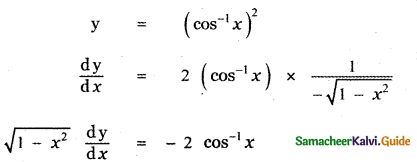Tamilnadu State Board New Syllabus Samacheer Kalvi 11th Maths Guide Pdf Chapter 10 Differentiability and Methods of Differentiation Ex 10.4 Text Book Back Questions and Answers, Notes.

## Tamilnadu Samacheer Kalvi 11th Maths Solutions Chapter 10 Differentiability and Methods of Differentiation Ex 10.4

Find the derivatives of the following:

Question 1.
y = xcos x
y = xcos x
Taking log on both sides
log y = log xcos x
log y = cos x log x
Differentiating with respect to x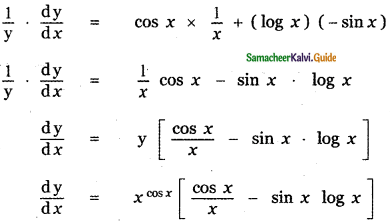Question 2.
y = xlog x + (log x)x
y = xlog x + (log x)x
Let u = xlog x, v = (log x)x
log u = log xlog x
log u = (log x) (log x)
log u = (log x)2v = (log x)x
log v = log (log x)x
log v = x log (log x)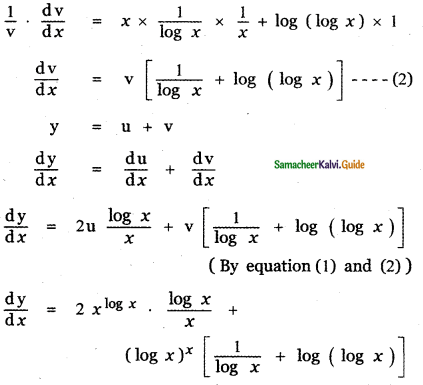Question 3.
$$\sqrt{x y}$$ = e(x – y)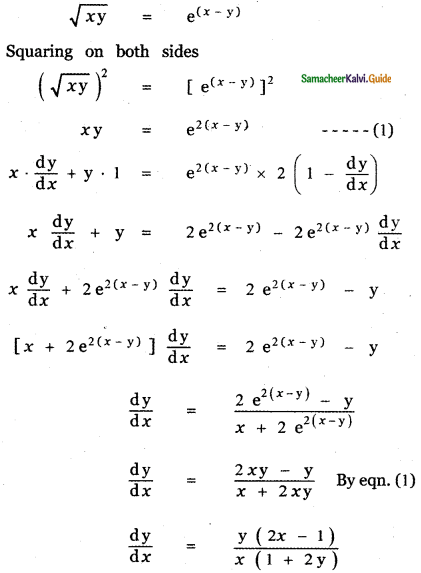Question 4.
xy = yx
xy = yx
Taking log on both sides
log xy = log yx
y log x = x log y
Differentiate with respect to xQuestion 5.
(cos x)log x
y = (cos x)log x
Taking log on both sides
log y = log (cos x)log x
log y = (log x) log (cos x)
Differentiating with respect to x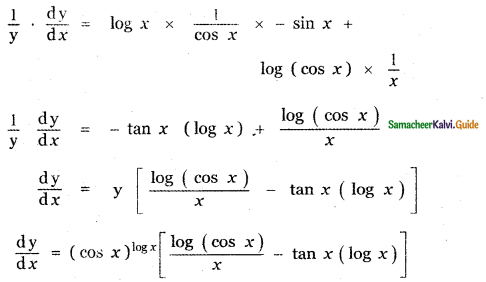Question 6.
$$\frac{x^{2}}{a^{2}}+\frac{y^{2}}{b^{2}}=1$$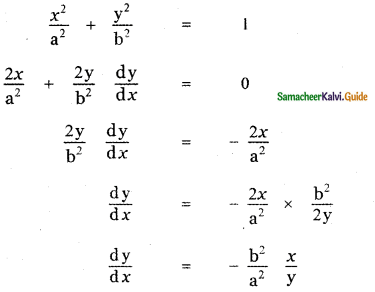Question 7.
$$\sqrt{x^{2}+y^{2}}=\tan ^{-1}\left(\frac{y}{x}\right)$$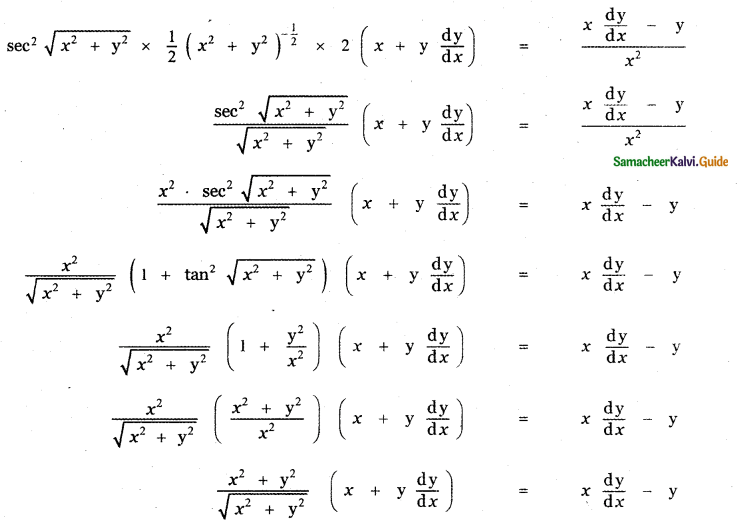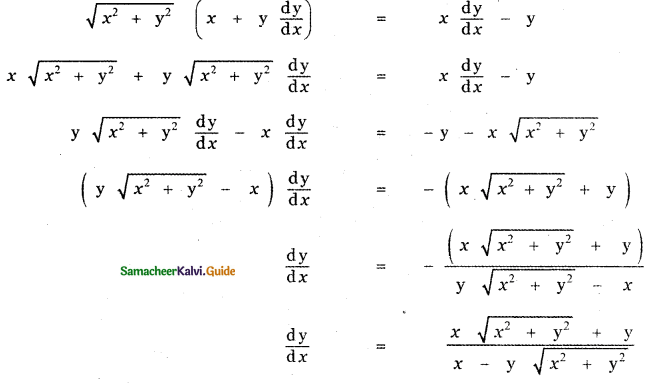Question 8.
tan (x + y) + tan (x – y) = x
tan (x + y) + tan (x – y) = x
Differentiating with respect to xQuestion 9.
If cos(xy) = x, show that
$$\frac{d y}{d x}=\frac{-(1+y \sin (x y))}{x \sin x y}$$
cos (xy) = x
Differentiating with respect to xQuestion 10.
$$\tan ^{-1} \sqrt{\frac{1-\cos x}{1+\cos x}}$$
Let y = $$\tan ^{-1} \sqrt{\frac{1-\cos x}{1+\cos x}}$$
[1 – cos 2θ = 2 sin2θ and 1 + cos 2θ = 2 sin2 θ]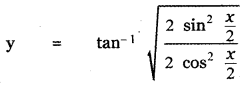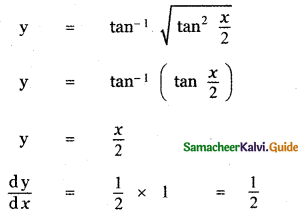Question 11.
tan-1 = $$\left(\frac{6 x}{1-9 x^{2}}\right)$$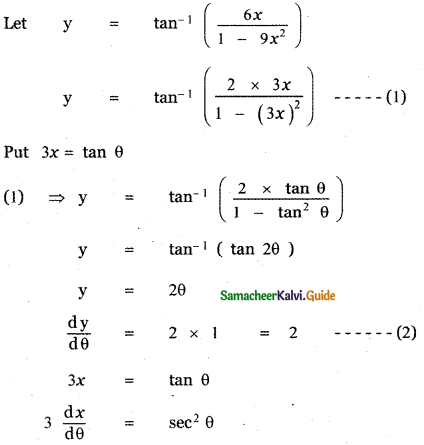Question 12.
$$\cos \left(2 \tan ^{-1} \sqrt{\frac{1-x}{1+x}}\right)$$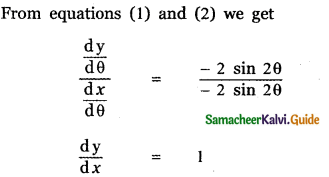Question 13.
x = a cost ; y = a sin3t
x = a cost , y = a sin3tQuestion 14.
x = a (cos t + t sin t);
y = a (sin t – t cos t)
x = a (cos t + t sin t) , y = a (sin t – t cos t)
$$\frac{d x}{d t}$$ = a [- sin t + t cos t + sin t ]
$$\frac{d x}{d t}$$ = at cos t —— (1)
y = a (sin t – t cos t)
$$\frac{d x}{d t}$$ = a [cos t – (t × – sin t + cos t × 1)]
$$\frac{d x}{d t}$$ = a[cos t + t sin t – cos t]
$$\frac{d x}{d t}$$ = at sin t —— (2)
From equations (1) and (2) we get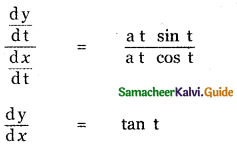Question 15.
x = $$\frac{1-t^{2}}{1+t^{2}}$$ , y = $$\frac{2 t}{1+t^{2}}$$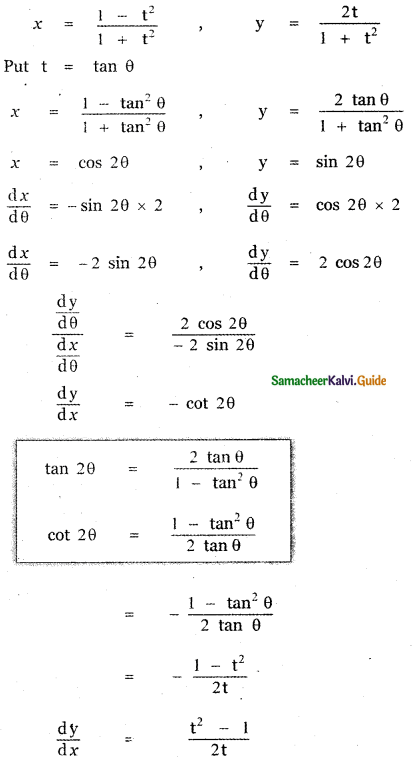Question 16.
cos-1$$\left(\frac{1-x^{2}}{1+x^{2}}\right)$$
Let y = cos-1$$\left(\frac{1-x^{2}}{1+x^{2}}\right)$$
Put x = tan θ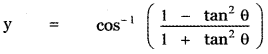y = cos-1 (cos 2θ)
y = 2θ
y = 2 tan-1 xQuestion 17.
sin-1 (3x – 4x3)
Let y = sin-1 (3x – 4x3)
Put x = sin θ
y = sin-1 (3 sin θ – 4 sin3 θ)
y = sin-1 (sin 3θ)
y = 3θ
y = 3 sin-1 x
$$\frac{d y}{d x}=\frac{3}{\sqrt{1-x^{2}}}$$

Question 18.
tan-1 $$\left(\frac{\cos x+\sin x}{\cos x-\sin x}\right)$$
Let y = tan-1 $$\left(\frac{\cos x+\sin x}{\cos x-\sin x}\right)$$Question 19.
Find the derivative of sin x2 with respect to x2.
Let u = sin x2
$$\frac{\mathrm{d} \mathrm{u}}{\mathrm{d} x}$$ = cos (x2) × 2x
$$\frac{\mathrm{d} \mathrm{u}}{\mathrm{d} x}$$ = 2x cos (x2)
Let v = x2Question 20.
Find the derivative of sin-1$$\left(\frac{2 x}{1+x^{2}}\right)$$ with respect to tan-1 x.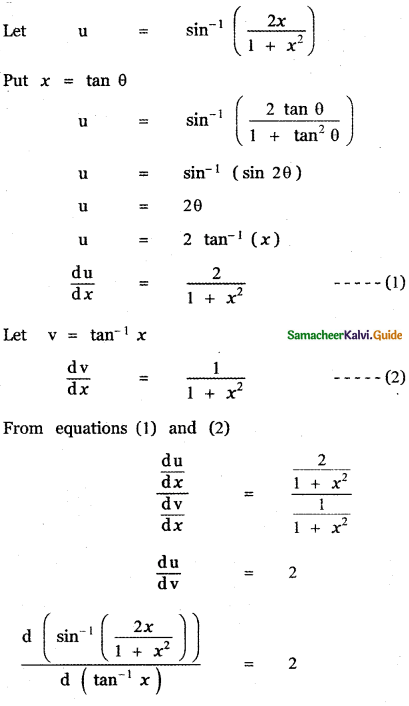Question 21.
If u = tan-1$$\frac{\sqrt{1+x^{2}}-1}{x}$$ and v = tan-1x, find $$\frac{\mathrm{du}}{\mathrm{dv}}$$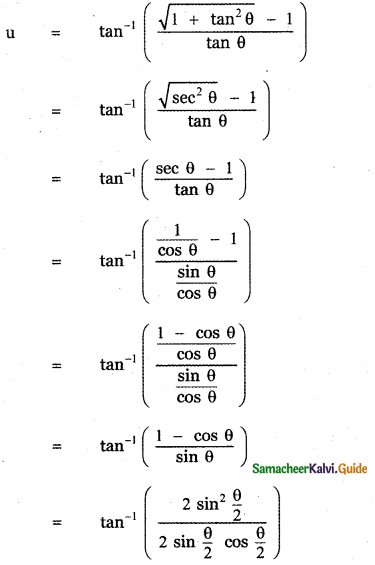Question 22.
Find the derivative with tan-1$$\left(\frac{\sin x}{1+\cos x}\right)$$ with respect to tan-1$$\left(\frac{\cos x}{1+\sin x}\right)$$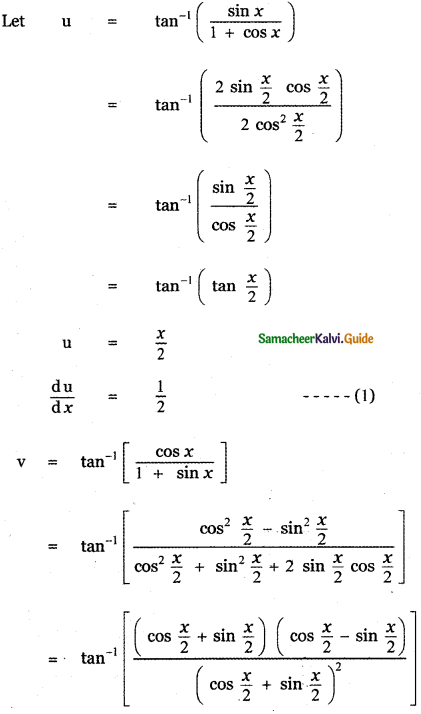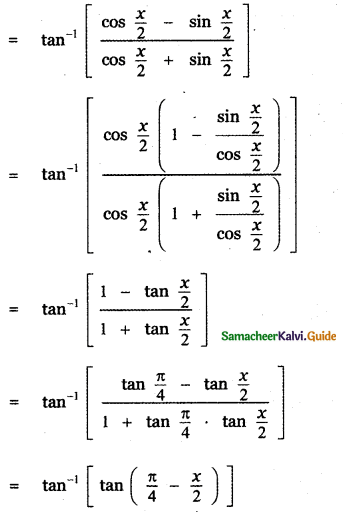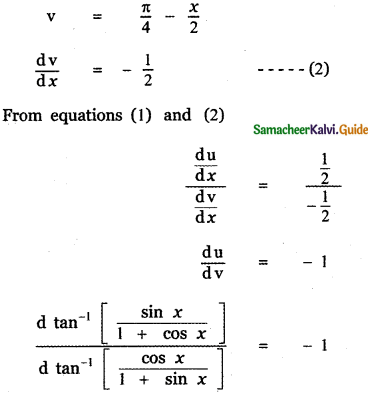Question 23.
If y = sin-1x then find y”.
y = sin-1 x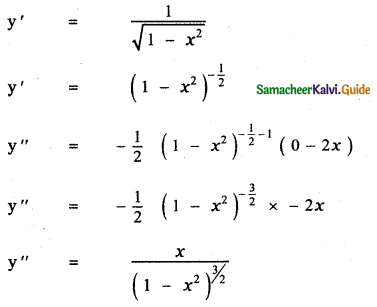Question 24.
If y = etan-1x, show that (1 + x2) y” + (2x – 1) y’ = 0
y = etan-1x
y = etan-1x $$\left(\frac{1}{1+x^{2}}\right)$$
⇒ y’ = $$\frac{y}{1+x^{2}}$$ ⇒ y'(1 + x2) = y
differentiating w.r.to x
y’ (2x) + (1 + x2) (y”) = y’
(i.e.) (1 + x2) y” + y’ (2x) – y’ = 0
(i.e.) (1 + x2) y” + (2x – 1) y’ = 0

Question 25.
If y = $$\frac{\sin ^{-1} x}{\sqrt{1-x^{2}}}$$, show that (1 – x2)y2 – 3xy1 – y = 0.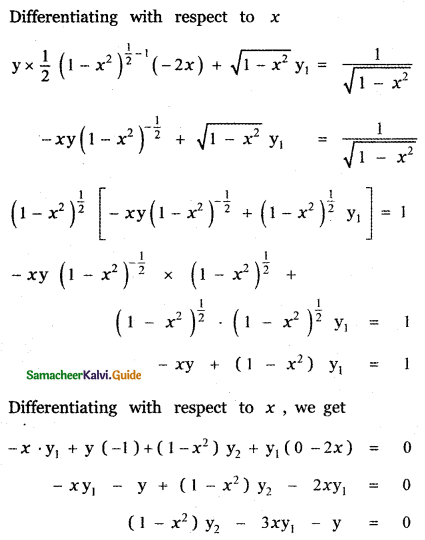Question 26.
If x = a (θ + sin θ), y = a (1 – cos θ) then prove that at θ = $$\frac{\pi}{2}$$, y” = $$\frac{1}{a}$$Question 27.
If sin y = x sin (a + y), the prove that $$\frac{d y}{d x}=\frac{\sin ^{2}(a+y)}{\sin a}$$, a ≠ nπ
cos y $$\frac{\mathrm{dy}}{\mathrm{d} x}$$ = x cos (a + y) (0 + $$\frac{\mathrm{dy}}{\mathrm{d} x}$$) + sin (a + y) . 1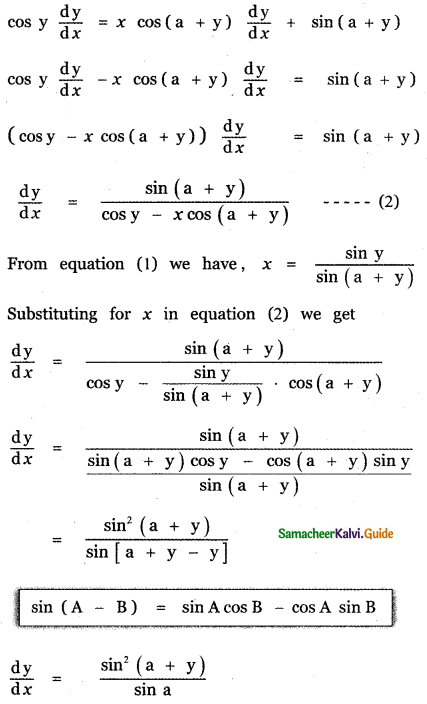If y = (cos-1 x)2, prove that (1 – x2) $$\frac{\mathrm{d}^{2} \mathrm{y}}{\mathrm{d} x^{2}}$$ – x $$\frac{\mathrm{dy}}{\mathrm{d} x}$$ – 2 = 0. Hence find y2 when x = 0.